## Example Questions

← Previous 1 3 4 5 6

### Example Question #1 : How To Find Research Summary In Chemistry

Both gases and liquids are considered to be fluids that have individual molecules that move around with kinetic and potential energy. Kinetic energy, defined as the energy related to motion, takes three forms: translational energy that occurs as a molecule moves from position A to position B, rotational energy that occurs as a molecule spins around an imaginary axis at its center of mass, and vibrational energy that occurs as individual atoms in a molecular bond move towards and away from each other. Usually, molecules possess varying combinations of kinetic energy forms. In contrast, potential energy is defined as stored energy that could be released to become kinetic energy. The total energy of a molecule is fixed, meaning that a molecule has some combination of kinetic and potential energies.

Varying amount of kinetic and potential energies define how molecules in a fluid interact with each other. For example, when the kinetic energy of a molecule is high (greater than 1000J), it can no longer interact with neighboring molecules strongly enough to remain a liquid. However, if the potential energies are too high (greater than 1000 J), molecules cannot escape a liquid to become a gas. If the kinetic energy is high and the potential energy is low, molecules tend to become a gas and can be modeled by an equation known as the Ideal Gas Law: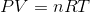Where P is the pressure of a gas, V is the volume, n is the number of moles of a gas, R is a constant, and T is temperature in degrees Kelvin.

The Ideal Gas Law perfectly applies to particles with no mass, no intermolecular interactions, and no true volume. However, real molecules do not adhere perfectly to the Ideal Gas Law.

In an oxygen molecule, O2, the two oxygen atoms oscillate about a fixed central point. Which form of kinetic energy is best described here?

Vibrational

None of the Above

Translational

Rotational

Vibrational

Explanation:

In the first paragraph, the passage tells us that individual atoms in a bond that move towards and away from each other constitute vibrational energy. Based on the question, the two oxygen atoms oscillate around the central point (the mid-point of their bond). Thus, this is vibrational energy.

### Example Question #1 : How To Find Research Summary In Chemistry

Both gases and liquids are considered to be fluids that have individual molecules that move around with kinetic and potential energy. Kinetic energy, defined as the energy related to motion, takes three forms: translational energy that occurs as a molecule moves from position A to position B, rotational energy that occurs as a molecule spins around an imaginary axis at its center of mass, and vibrational energy that occurs as individual atoms in a molecular bond move towards and away from each other. Usually, molecules possess varying combinations of kinetic energy forms. In contrast, potential energy is defined as stored energy that could be released to become kinetic energy. The total energy of a molecule is fixed, meaning that a molecule has some combination of kinetic and potential energies.

Varying amount of kinetic and potential energies define how molecules in a fluid interact with each other. For example, when the kinetic energy of a molecule is high (greater than 1000J), it can no longer interact with neighboring molecules strongly enough to remain a liquid. However, if the potential energies are too high (greater than 1000 J), molecules cannot escape a liquid to become a gas. If the kinetic energy is high and the potential energy is low, molecules tend to become a gas and can be modeled by an equation known as the Ideal Gas Law:Where P is the pressure of a gas, V is the volume, n is the number of moles of a gas, R is a constant, and T is temperature in degrees Kelvin.

The Ideal Gas Law perfectly applies to particles with no mass, no intermolecular interactions, and no true volume. However, real molecules do not adhere perfectly to the Ideal Gas Law.

An oxygen molecule moving from the left side of a chamber to the right would display what type(s) of kinetic energy?

Translational

Rotational

Vibrational

All of the Above

All of the Above

Explanation:

In the first passage, after describing the different types of kinetic energy, the passage notes that a molecule would likely have varying combinations of kinetic energy. Thus, a molecule moving across the room would display translational, vibrational, and rotational motion.

### Example Question #881 : Act Science

Both gases and liquids are considered to be fluids that have individual molecules that move around with kinetic and potential energy. Kinetic energy, defined as the energy related to motion, takes three forms: translational energy that occurs as a molecule moves from position A to position B, rotational energy that occurs as a molecule spins around an imaginary axis at its center of mass, and vibrational energy that occurs as individual atoms in a molecular bond move towards and away from each other. Usually, molecules possess varying combinations of kinetic energy forms. In contrast, potential energy is defined as stored energy that could be released to become kinetic energy. The total energy of a molecule is fixed, meaning that a molecule has some combination of kinetic and potential energies.

Varying amount of kinetic and potential energies define how molecules in a fluid interact with each other. For example, when the kinetic energy of a molecule is high (greater than 1000J), it can no longer interact with neighboring molecules strongly enough to remain a liquid. However, if the potential energies are too high (greater than 1000 J), molecules cannot escape a liquid to become a gas. If the kinetic energy is high and the potential energy is low, molecules tend to become a gas and can be modeled by an equation known as the Ideal Gas Law:Where P is the pressure of a gas, V is the volume, n is the number of moles of a gas, R is a constant, and T is temperature in degrees Kelvin.

The Ideal Gas Law perfectly applies to particles with no mass, no intermolecular interactions, and no true volume. However, real molecules do not adhere perfectly to the Ideal Gas Law.

The relationship between total energy, kinetic energy, and potential energy could best be described as: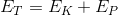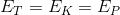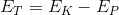Explanation:

The end of the first paragraph helps us answer this question. We are told that a molecule contains a fixed amount of total energy and that some combination of kinetic and potential energy combines to create this sum total. Thus, the relationship that best depicts how the various forms of energy relate is.

### Example Question #1 : How To Find Research Summary In Chemistry

Both gases and liquids are considered to be fluids that have individual molecules that move around with kinetic and potential energy. Kinetic energy, defined as the energy related to motion, takes three forms: translational energy that occurs as a molecule moves from position A to position B, rotational energy that occurs as a molecule spins around an imaginary axis at its center of mass, and vibrational energy that occurs as individual atoms in a molecular bond move towards and away from each other. Usually, molecules possess varying combinations of kinetic energy forms. In contrast, potential energy is defined as stored energy that could be released to become kinetic energy. The total energy of a molecule is fixed, meaning that a molecule has some combination of kinetic and potential energies.

Varying amount of kinetic and potential energies define how molecules in a fluid interact with each other. For example, when the kinetic energy of a molecule is high (greater than 1000J), it can no longer interact with neighboring molecules strongly enough to remain a liquid. However, if the potential energies are too high (greater than 1000 J), molecules cannot escape a liquid to become a gas. If the kinetic energy is high and the potential energy is low, molecules tend to become a gas and can be modeled by an equation known as the Ideal Gas Law:Where P is the pressure of a gas, V is the volume, n is the number of moles of a gas, R is a constant, and T is temperature in degrees Kelvin.

The Ideal Gas Law perfectly applies to particles with no mass, no intermolecular interactions, and no true volume. However, real molecules do not adhere perfectly to the Ideal Gas Law.

As the kinetic energy of a molecule increases, one would expect the potential energy to:

Increase

Remain the Same

Decrease

Cannot Be Determined

Decrease

Explanation:

Using the last few sentences of the first paragraph as a guide, we can see that the total energy is fixed. Thus, some combination of kinetic and potential energies makes up the total energy. We can relate how the energies combine with the following formula:. Thus, if the kinetic energy increases, the potential energy would have to decrease to keep the sum total the same.

### Example Question #5 : How To Find Research Summary In Chemistry

Both gases and liquids are considered to be fluids that have individual molecules that move around with kinetic and potential energy. Kinetic energy, defined as the energy related to motion, takes three forms: translational energy that occurs as a molecule moves from position A to position B, rotational energy that occurs as a molecule spins around an imaginary axis at its center of mass, and vibrational energy that occurs as individual atoms in a molecular bond move towards and away from each other. Usually, molecules possess varying combinations of kinetic energy forms. In contrast, potential energy is defined as stored energy that could be released to become kinetic energy. The total energy of a molecule is fixed, meaning that a molecule has some combination of kinetic and potential energies.

Varying amount of kinetic and potential energies define how molecules in a fluid interact with each other. For example, when the kinetic energy of a molecule is high (greater than 1000J), it can no longer interact with neighboring molecules strongly enough to remain a liquid. However, if the potential energies are too high (greater than 1000 J), molecules cannot escape a liquid to become a gas. If the kinetic energy is high and the potential energy is low, molecules tend to become a gas and can be modeled by an equation known as the Ideal Gas Law:Where P is the pressure of a gas, V is the volume, n is the number of moles of a gas, R is a constant, and T is temperature in degrees Kelvin.

The Ideal Gas Law perfectly applies to particles with no mass, no intermolecular interactions, and no true volume. However, real molecules do not adhere perfectly to the Ideal Gas Law.

As the potential energy of a molecule decreases, one would expect the kinetic energy to:

Decrease

Remain the Same

Increase

Cannot Be Determined

Increase

Explanation:

Using the last few sentences of the first paragraph as a guide, we can see that the total energy of a molecule is fixed and contains a fixed combination of kinetic and potential energies. Thus, we can relate the combination to the total by the following formula:. Thus, if the potential energy decreases, the kinetic energy must increase in order to keep the total energy of the molecule constant.

### Example Question #6 : How To Find Research Summary In Chemistry

Both gases and liquids are considered to be fluids that have individual molecules that move around with kinetic and potential energy. Kinetic energy, defined as the energy related to motion, takes three forms: translational energy that occurs as a molecule moves from position A to position B, rotational energy that occurs as a molecule spins around an imaginary axis at its center of mass, and vibrational energy that occurs as individual atoms in a molecular bond move towards and away from each other. Usually, molecules possess varying combinations of kinetic energy forms. In contrast, potential energy is defined as stored energy that could be released to become kinetic energy. The total energy of a molecule is fixed, meaning that a molecule has some combination of kinetic and potential energies.

Varying amount of kinetic and potential energies define how molecules in a fluid interact with each other. For example, when the kinetic energy of a molecule is high (greater than 1000J), it can no longer interact with neighboring molecules strongly enough to remain a liquid. However, if the potential energies are too high (greater than 1000 J), molecules cannot escape a liquid to become a gas. If the kinetic energy is high and the potential energy is low, molecules tend to become a gas and can be modeled by an equation known as the Ideal Gas Law:Where P is the pressure of a gas, V is the volume, n is the number of moles of a gas, R is a constant, and T is temperature in degrees Kelvin.

The Ideal Gas Law perfectly applies to particles with no mass, no intermolecular interactions, and no true volume. However, real molecules do not adhere perfectly to the Ideal Gas Law.

The relationship between kinetic and potential energy may best be illustrated by: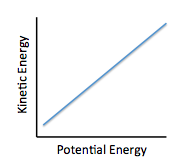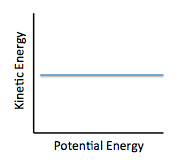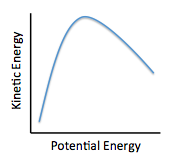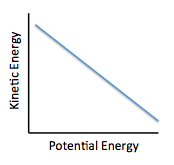Explanation:

The first paragraph helps us understand that a total amount of energy is fixed per molecule and is a combination of kinetic and potential energies. We can relate the two energies by the following formula:. Thus, as kinetic energy decreases, potential energy increases. The only curve that shows this relationship is a linear curve with a negative slope.

### Example Question #7 : How To Find Research Summary In Chemistry

Chemists can model how solids, liquids, and gases behave at different temperatures and pressures with a graph called a phase diagram. When the pressure and temperature are simultaneously known, a scientist can predict whether the material will be in a specific state. The diagram is divided into sections depending on the phase and the lines between sections represent phase transitions occurring between two or more separate phases.

In general, solids of neatly stacked molecules exist when temperatures are low and pressures are intermediate. These values decrease the kinetic energy of the molecules enough to allow for attractive forces to begin the stacking process. Liquids, by contrast, are found at intermediate pressures and temperatures. The temperature is high enough to impart enough kinetic energy to prevent solid formation and the pressure is high enough to prevent the liquid from becoming a gas. Finally, a gas forms at low pressures and high temperatures. The high level of kinetic energy prevents molecules from associating with one another.

Materials can undergo processes called phase transitions, meaning they can transition from one phase to another. The transition from a solid to a liquid is called melting, while the reverse transition is called freezing. Vaporization occurs when a liquid becomes a gas, while condensation occurs when a gas becomes a liquid. Finally, in a process called sublimation, a solid can directly become a gas without passing through a liquid phase. Additionally, when a gas directly becomes a solid, this is known as deposition.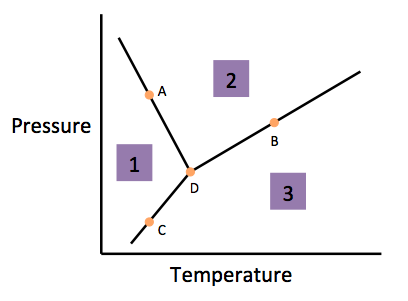In order to predict the state of a material, the scientist must know __________.

Pressure

Neither Temperature Nor Pressure

Temperature

Both Temperature and Pressure

Both Temperature and Pressure

Explanation:

The passage states that a scientist must know both temperature and pressure in order to find where in the phase diagram a material is. Without this knowledge, the scientist cannot determine whether the material is in a solid, liquid, or gaseous state.

### Example Question #8 : How To Find Research Summary In Chemistry

The Millikin oil drop experiment is among the most important experiments in the history of science.  It was used to determine one of the fundamental constants of the universe, the charge on the electron. For his work, Robert Millikin won the Nobel Prize in Physics in 1923.

Millikin used an experimental setup as follows in Figure 1. He opened a chamber of oil into an adjacent uniform electric field.  The oil droplets sank into the electric field once the trap door opened, but were then immediately suspended by the forces of electricity present in the field.

Figure 1: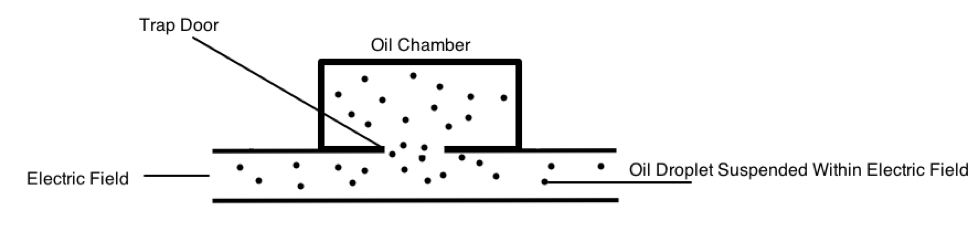By determining how much force was needed to exactly counteract the gravity pulling the oil droplet down, Millikin was able to determine the force of electricity.  This is depicted in Figure 2.

Using this information, he was able to calculate the exact charge on an electron.  By changing some conditions, such as creating a vacuum in the apparatus, the experiment can be modified.

Figure 2: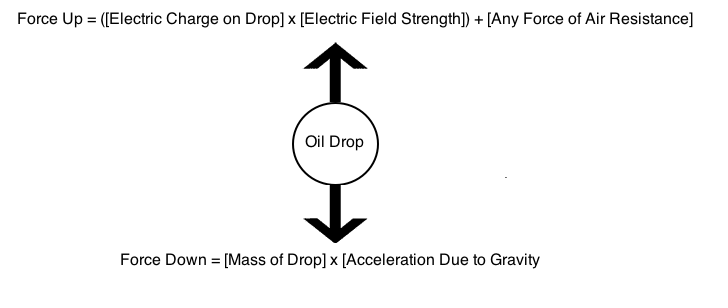When the drop is suspended perfectly, the total forces up equal the total forces down.  Because Millikin knew the electric field in the apparatus, the force of air resistance, the mass of the drop, and the acceleration due to gravity, he was able to solve the following equation: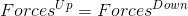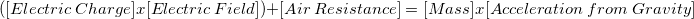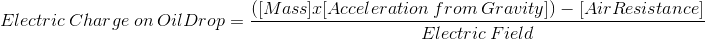Table 1 summarizes the electric charge found on oil drops in suspension.  Millikin correctly concluded that the calculated charges must all be multiples of the fundamental charge of the electron.  A hypothetical oil drop contains some net charge due to lost electrons, and this net charge cannot be smaller than the charge on a single electron.

Table 1:

 Trial # Electric Charge Calculated in Coulombs (C) Vacuum Used? 1 1.602176487 x 10-8 No 2 1.602176487 x 10-2 Yes 3 1.602176487 x 10-6 No 4 1.602176487 x 10-4 Yes

Which of the following statements is most consistent with the findings of this experiment?

An individual electron may have any charge, as long as it is a multiple of the fundamental charge

The fundamental charge on an electron varies with time

An individual electron may have any charge, as long as it is a factor of the fundamental charge

It is impossible to predict the fundamental charge on an electron

All electrons have identical values for their fundamental charges

All electrons have identical values for their fundamental charges

Explanation:

The data presented in the passage suggest that the fundamental charge on an electron must be a factor of the observed charge on the entire oil drop.  While this value can change, the fundamental charge on all electrons will be identical.

### Example Question #1 : How To Find Research Summary In Chemistry

Current high levels of fossil fuel use, including coal-burning power plants and gasoline-powered automobiles, have helped contribute to the high concentrations of sulfur trioxide, SO3, found in the atmosphere. When sulfur trioxide and water interact, they can undergo the following chemical reaction to produce sulfuric acid, which is the main contributor to acid rain worldwide: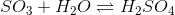Acid rain showers are particularly common near coal-burning power plants and large cities. These showers are responsible for significant economic damage to sidewalks, roads, and buildings. Scientists interested in studying the effects of acid rain often use basic substances like calcium carbonate, the main component of limestone buildings, and expose them to varying volumes of acid rain to determine what volume of acid rain is necessary to begin to erode a building. A sample graph of one scientist’s experiment is replicated below: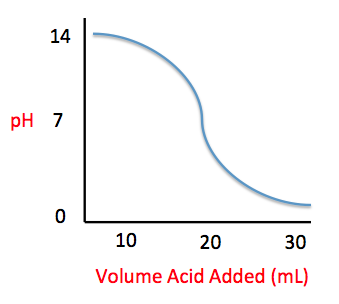Measuring acid and base levels is commonly done with a scale called pH, which uses the concentration of hydrogen ions to determine the acidity. Hydrogen ions are in a balance with hydroxide ions to give a scale with a range from 0 to 14. Values equal to or between 0 and 6.9 represent the acidic range where hydrogen ions predominate and values equal to or ranging from 7.1 and 14 represent the basic range where hydroxide ions predominate. Thus, the more hydrogen ions present, the more acidic the solution.

Scientists can tell when a titration (pH) experiment passes a certain pH using compounds called indicators. Indicators are usually colorless at pH levels below that of their specified color change. A table of indicators used by the above scientists and the pH at which they change colors is presented below.The relationship between the concentrations of hydrogen and hydroxide ions in an acid-base solution may best be illustrated as which of the following?

Cannot Be Determined

Inverse

Direct

Indirect

Inverse

Explanation:

According to the paragraph below the passage graph, the number of hydrogen ions present in a solution is measured by a pH scale. The end of the paragraph tells us that when pH is low, the number of hydrogen ions is high and the number of hydroxide ions in low. In other words, when one is high, the other is low. This describes a reciprocal relationship.

### Example Question #883 : Act Science

A student wants to perform an experiment that tests the relationship between the pressure of a gas and the volume it occupies. To perform this experiment, the student places a specific type of gas in a sealed chamber that can change pressure and that can adapt its volume to the gas within it. The chamber also adjusts to the changing pressure such that the temperature (which also has an effect on gas volume) does not change. The following data was obtained: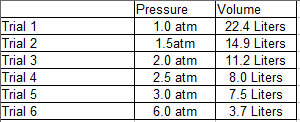In Trial 4, the chamber used in the experiment malfunctioned and there was a slight decrease in temperature, leading to a faulty reading of volume for Trial 4. Given this information, what can we say about the relationship between the temperature of a gas and its volume?

Temperature and volume are directly related.

Temperature and volume are indirectly and linearly related.

Temperature and volume are directly and linearly related.

Temperature and volume are directly and exponentially related.

Temperature and volume are indirectly and exponentially related.